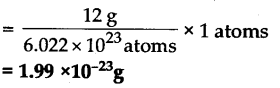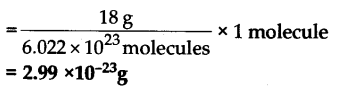# Calculate the mass of: (a) 1 atom of carbon (b) 0.02 mole of sodium (c) 1 molecule of water

(a) We know that, 1 mole of carbon atoms = Gram atomic mass of carbon = 12 g
Also, 1 mole of carbon atoms = 6.022 x 10^23 atoms of carbon.
Therefore
6.022 x 10^23 atoms of carbon weigh = 12 g
So, 1 atom of carbon weigh(b) We know that,
1 mole of sodium atoms = Gram atomic mass of sodium = 23 g
Mass of 0.02 mole of sodium = 0.02 x 23 g = 0.46 g

© 1 mole of H20 = Gram molecular mass of H20 = 1x2 +16 = 18 g
We know that, 1 mole of H2O
= 1 mole = 6.022 x 10^23 molecules
It means that, 6.022 x 10^23 molecules of water have mass = 18 g
Therefore
1 molecule of H20 have mass 18 g